# Logarithms

## Logarithms

We started our previous article on exponential equations with equation 2 x= 8. Everything was clear there: x = 3.

Now consider the equation 2 x= 7.

According to the graph of the function y = 2 xwe see that this equation has a root, and, moreover, the only one.It is clear that this root is not an integer (since 2 2= 4, 2 3= 8). Moreover, it turns out that it is not even a rational number, that is, it cannot be represented as an ordinary fraction. Intuitively, we only feel that it is less than 3, but not much.

This root is denoted log 27 (reads: “logarithm base two.” It is an irrational number, that is, an infinite non-periodic decimal fraction. The calculator gives: log 27 = 2.807354922057604107 ...

So, our number is log 27 is an exponent to which 2 must be raised to get 7.

We now give a general definition of the logarithm. Let a> 0 and a ≠ 1 (the conditions are the same as for the base of the exponential function).

Definition. Base a logarithm of a positive number b (denoted by log ab) is the exponent to which a must be raised to get b.

In other words,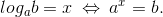For instance:because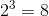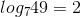, because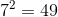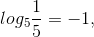because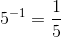;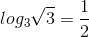, because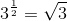.

Logarithm base 10 is called decimal and is denoted by lg. For example, lg 100 = 2, lg 1000 = 3, lg 0.01 = −2.

The logarithm with base e is called natural and is denoted by ln.

Please note: the logarithm is only defined for positive numbers. The reason is that the exponential function can only take positive values. For example, the number log 2(−4) does not exist: no matter how much we raise 2, we will never get −4.

Do not forget also about the restrictions on the base of the logarithm: 0 <a <1 or a> 1.

Basic formulas

By definition, log ab is the exponent to which the number a must be raised to get the number b:

Formula (1) is called basic logarithmic identity Here is another way to write the basic logarithmic identity:

log aax= x.

Let us list the properties of logarithms. They are simple consequences of the power rules. All logarithms below are considered definite.

The logarithm of the product is the sum of the logarithms:

 log a(bc) = log ab + log ac. (2)

The logarithm of the quotient is the difference between the logarithms:$log_ {a} \ frac {b} {c} = log_ {a} b-log_ {a} c$ (3)

The exponent of the logarithm "jumps" in front of the logarithm: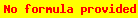$log_ {a} b ^ {m} = mlog_ {a} b$ (four)

The exponent of the base of the logarithm also "jumps", but in the form of an inverse number:$log_ {a ^ {n}} b = \ frac {1} {n} log_ {a} b$ (five)

Formulas (4) and (5) together give:(6)

In particular, if m = n, we get the formula: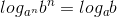(7)

For instance,.

Finally, the most important formula for the transition to a new foundation: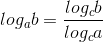(8)

In particular, if c = b, then log bb = 1, and then:(9)

Here are some examples from the job bank. one.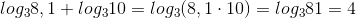(applied formula (2) the sum of logarithms).

2.(applied the basic logarithmic identity (1))

3.$log ^ {2} _ {\ sqrt {7}} 49 = (log _ {\ sqrt {7}} 49) ^ {2} = (log _ {\ sqrt {7}} 7 ^ {2}) ^ {2} = (2log _ {\ sqrt {7}} 7) ^ {2} = (2 \ cdot 2) ^ {2} = 16$(we applied formula (4).

four.$log_ {0.8} 3 \ cdot log_ {3} 1.25 = log_ {0.8} 3 \ cdot \ frac {log_ {0.8} 1.25} {log_ {0.8} 3} = log_ {0.8} 1.25 = log _ {\ frac {4} {5}} \ frac {5} {4} = - 1$(applied formula (9), passing to a new base 0.8).

five.$\ frac {9 ^ {log_ {5} 50}} {9 ^ {log_ {5} 2}} = 9 ^ {log_ {5} 50-log_ {5} 2} = 9 ^ {log_ {5} 25} = 9 ^ {2} = 81$(applied formula (3) difference of logarithms)

A bit of history

Now you understand what logarithms are and how to use them. But what are they for? Or is it just a math toy with clever instructions for use?

The concept of a logarithm and logarithmic tables appeared in the 17th century, and their significance was enormous.

These days, calculations are not difficult - everyone has a calculator. And what was considered in "pre-computer" times?

It was possible to add and subtract on the abacus, but to multiply and divide "in a column" was slow and difficult.

In the 15-17th centuries, in the era of great geographical discoveries, trade, economics and science began to develop rapidly. The requirements for mathematics grew: the calculations became more complex, and the accuracy - for example, for solving navigation problems - was needed more and more.

A tool was needed to simplify and speed up the calculations, and logarithms were such a tool.

Suppose b and c are large numbers that need to be multiplied. The advent of tables of logarithms (for example, with base 10) greatly simplified this task. Now it was enough for the calculator to find the decimal logarithms of the numbers b and c from the tables, add them (on the abacus) and get the logarithm of the product: lgb + lgc = lg (bc).

And then, using the table of logarithms, find the very product of the numbers b and c.

No wonder the French mathematician and astronomer Laplace said that the invention of logarithms lengthened the life of calculators. The slide rule (which engineers used until the 70s of the twentieth century) was no less progressive invention than the modern calculator.

But that is not all! We would not deal with logarithms if they had only historical, "museum" value. We will talk about unexpected applications of logarithms in the next article on the logarithmic function.

Any significant logarithmic problem cannot be solved without knowing the special rules of logarithms. Or rather, the main properties. Fortunately, there are not many of these properties and it will not be difficult to learn them. But you need to know them both from left to right and in the opposite direction.

### Let's consider individual properties in more detail:

• Logarithmic zero. An elementary property that must be remembered. Whatever the base of the logarithm, if the argument is 1, then the logarithm is always 0.
• Logarithmic unit. Another simple property: if the argument and the base of the logarithm are the same, then the value of the logarithm will be equal to one.
• Basic logarithmic identity. Excellent property that turns a four-story expression into a very basic b. The essence of this formula: the base a, raised to the power of the logarithm with the base a, will be equal to b.
• Sum of logarithms. When multiplying logarithm numbers, you can make of them the sum of 2 logarithms, which will have the same base. And so the incalculable logarithms become simple.
• Logarithm of the quotient. Here the situation is similar to the sum of logarithms. When dividing numbers, we get the difference of two logarithms with the same base.
Not every student can afford to spend a semester at a university 100 000 ₽ ... But it's cool that there is grants to study. Grant-na-vuz.rf this is the opportunity to study in the desired specialty. Link everyone will receive a bonus from 300 ₽ before 100 000 ₽ grant-at-university.rf
• Removing the exponent from the logarithm. As many as 3 rules apply here. It's simple: if the degree is at the base or argument of the logarithm, then it can be moved outside the logarithm, in accordance with these formulas:
• Formulas for the transition to a new base. They are needed for expressions with logarithms, which have different bases. Such formulas are mainly used to solve logarithmic inequalities and equations.